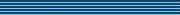| home      | people     | research     | publications    | seminars    | events     | contactCommunication Networks   | Systems Biology   | Hybrid Systems    | Machine Learning   | Dynamics & Interaction### Schools Mathematics Grand Challenge

This week's puzzles have geometry as a theme. Sometimes to solve a geometry problem it helps to look at it from a different angle (literally!) or to draw an extra line. Some facts that you may find useful.

1. Remember that the area of a triangle is half the length of the base times the height measured perpendicular to the base.
2. The three angles at the corners of a triangle always add up to 180 degrees.
3. An isosceles triangle has two sides the same length. The two angles opposite those two sides will also be the same.

PROBLEM 22:

John has been given a field in the shape of a triangle. Two sides of the triangle are exactly 10 metres long. What is the largest possible area, in square metres, of John's triangular field? Give your answer as the number of square metres. There is no need to include units.

PROBLEM 23:

Have a look at the following picture. The circle is area 438 units and has centre C. The line from A to E goes through the centre and the line from D to B goes through the centre. The angle between CA and AB is 60 degrees.If the area of the zig-zaggy triangle CDF is 30 units, what is the area of the bricked piece of the picture? Again, there is no need to give units.

PROBLEM 24:

In the triangle in the diagram below, the length of the side AB is the same as the length of the side AC. The point N divides the line from B to C into two equal parts. Also, the line BM divides the angle ABC into two equal parts, and the line from B to M is two times as long as the line from A to N.What is the size, in degrees, of the angle BAC (the largest angle) in the triangle?